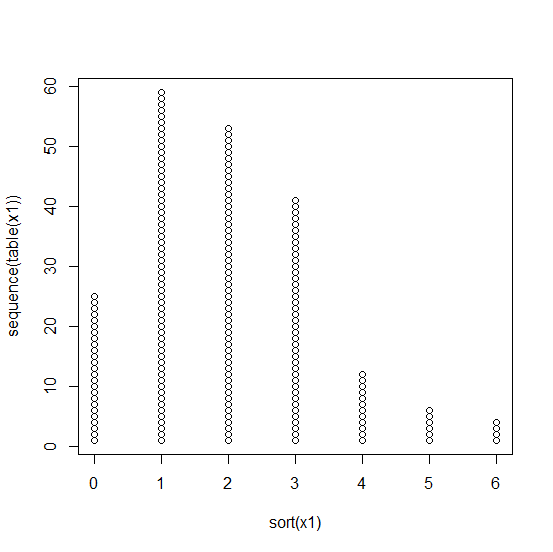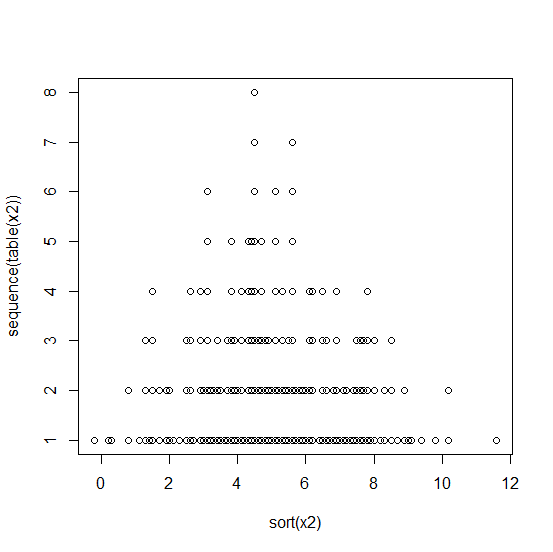# How to create a histogram with dots instead of bars in base R?

To create a histogram with dots instead of bars, we can use the plot function in base R. The x values will be based on the sorting of the vector values and the y values will be based on the sequence of the table for the vector values. Therefore, we would be needing sorting and table with sequence. Check out the below examples to understand how it can be done.

## Example1

> x1<-rpois(200,2)
> plot(sort(x1),sequence(table(x1)))

## Output## Example2

> x2<-round(rnorm(200,5,2),1)
> plot(sort(x2),sequence(table(x2)))

## Output